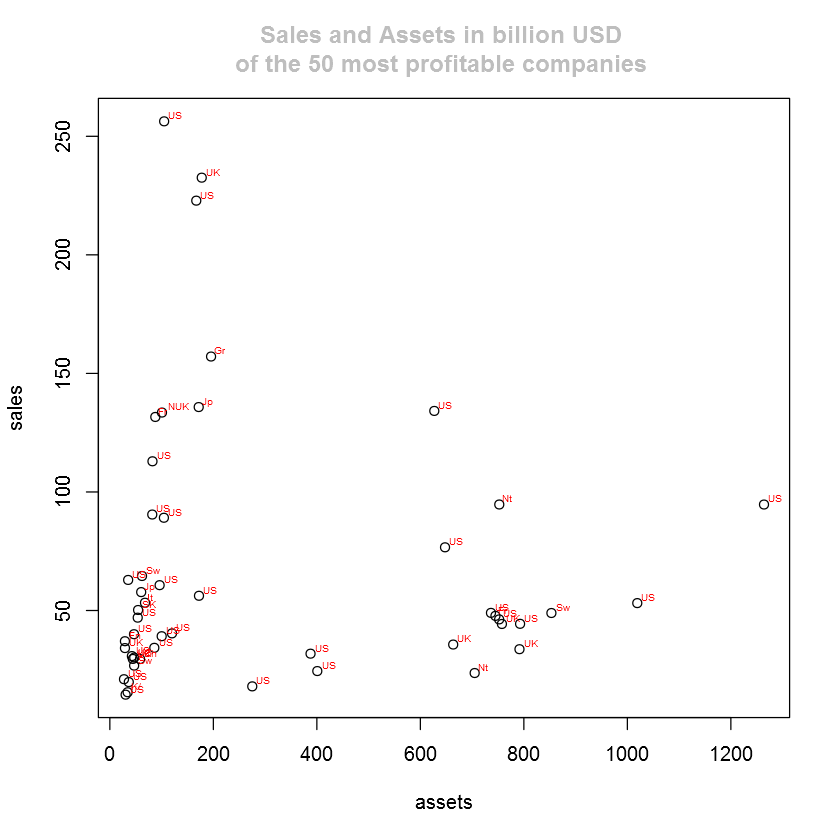# Fisseha Berhane, PhD

#### Data Scientist443-970-2353[email protected] CV Resume### World's Biggest Companies¶

Let's visualize the world's biggest companies using the Forbes2000 data from HSAUR2 package. First, we need to check if the package is installed else install it.

In :
if (!require(HSAUR2)){
install.packages('HSAUR2', repos='http://cran.us.r-project.org')}


In :
library(HSAUR2)


Then, we need to attach the Forbes2000 data.

In :
data(Forbes2000)


We can read the details of the data by using ?Forbes2000.

Let's see the variables using the names command.

In :
names(Forbes2000)

Out:
1. "rank"
2. "name"
3. "country"
4. "category"
5. "sales"
6. "profits"
7. "assets"
8. "marketvalue"

How many observations do we have?

In :
dim(Forbes2000)

Out:
1. 2000
2. 8

### Most profitable companies

Let's plot sales against assets for the 50 most profitable companies in the Forbes2000 data set.

To label each point with the appropriate country name in our plot, we will abbrevaite country names so that the plot will not be messy. To put country labels in our plot, we will install the "calibrate" package if it is not already installed.

In :
if (!require(calibrate)){
install.packages('calibrate', repos='http://cran.us.r-project.org')}


In :
library(calibrate)

In :
options(jupyter.plot_mimetypes = 'image/png') # I am using Jupyter and this command makes
# my plots inline

In :
profits_all = na.omit(Forbes2000$profits) # all_profts without No data order_profits = order(profits_all) # index of the profitable companies in decreasing order top_50 = rev(order_profits)[1:50] # top 50 profitable companies sales = Forbes2000$sales[top_50]       # sales of the 50 top profitable companies
assets = Forbes2000$assets[top_50] # assets of the 50 top profitable companies countries = Forbes2000$country[top_50] # countries where the 50 top profitable companies are found

plot(assets,sales,pch =1)
textxy(assets,sales, abbreviate(countries,2),col = "red",cex=0.5)  # used to put the countries where the companies are found
title(main = "Sales and Assets in billion USD \n of the 50 most profitable companies ", col.main = "gray")### Sales by countries¶

Let's calculate the average value of sales in billion USD for the companies in each country in the Forbes data set.

We can use the handy command tapply and calculate the mean easily by passing single line of command.

In :
meansales = tapply(Forbes2000$sales, Forbes2000$country, mean, na.rm = TRUE)


In the code above, for each level of the factor country, tapply determines the corresponding elements of the numeric vector sales and supply them to the mean function with additional argument na.rm = TRUE.

### Most profitable companies by country¶

Let's calculate the number of companies in each country with profits above 5 billion US dollars to see thier distributions.

To find the number of companies in each country with profit greater than 5 billion Us dollars, the indices of the profit data which are more than 5 billion US dollars are obtained and the countries of these indices are obtained. Then, finally a summary of the number of companies with profit greater than 5 billion US dollars in each country is tabulated.

In :
profitgt5 = which(Forbes2000$profits >5) # Get the indices of the companies with profit greater than 5 billion US dollars countries = Forbes2000$country               #  Get country names from the Forbes2000 data set
country_gt_5_profit = countries[profitgt5]   # Get the countries of the companies which have profit greater than 5 billion US dollars
in_each_country = table(country_gt_5_profit) # Get the number of companies with greater than 5 billion US dollars in each country

x = which(in_each_country>0)                 # To search the indices with non-zero values

profitables = in_each_country[x]             # Gives the number of companies in each country with profit greater than 5 billion US dollars
profitables

Out:
China
1
France
1
Germany
1
Japan
1
Netherlands/ United Kingdom
1
South Korea
1
Switzerland
3
United Kingdom
3
United States
20
In [ ]:
country=names(profitables)
companies=as.vector(profitables)

profitables=as.data.frame(list(country=country,companies=companies))

ggplot(profitables, aes(country,companies))+
geom_bar(stat='identity',fill='orange',color='black')+
ggtitle('Number of companies \nwith profits above \$5\n billion by country')+
theme(plot.title = element_text(size = 18,colour="blue"))+
theme(axis.title.x=element_blank(),axis.title.y =element_blank(),axis.text.y = element_text(colour="grey20",size=14,angle=0,hjust=1,vjust=0,face="plain"),axis.text.x = element_text(colour="grey20",size=14,angle=60,hjust=.5,vjust=.5,face="plain"))+coord_flip()

Out:As expected more than 60% of them are from the USA.